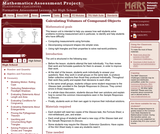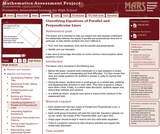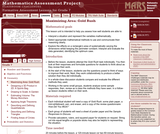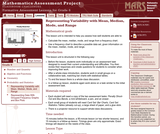Updating search results...

# 43 Results

View
Selected filters:
• MCCRS.Math.Practice.MP.1Conditional Remix & Share Permitted
CC BY-NC-SA
Rating
3.25 stars

This task was developed by high school and postsecondary mathematics and design/pre-construction educators, and validated by content experts in the Common Core State Standards in mathematics and the National Career Clusters Knowledge & Skills Statements. It was developed with the purpose of demonstrating how the Common Core and CTE Knowledge & Skills Statements can be integrated into classroom learning - and to provide classroom teachers with a truly authentic task for either mathematics or CTE courses.

Material Type:
Activity/Lab
Assessment
Homework/Assignment
Lesson Plan
Provider:
National Association of State Directors of Career Technical Education Consortium
Provider Set:
Career Technical Education
Date Added:
03/05/2012Only Sharing Permitted
CC BY-NC-ND
Rating
0.0 stars

This lesson unit is intended to help teahcers assess how well students solve problems involving measurement, and in particular, to identify and help students who have the following difficulties; computing measurements using formulas; decomposing compound shapes into simpler ones; using right triangles and their properties to solve real-world problems.

Material Type:
Assessment
Lesson Plan
Provider:
Shell Center for Mathematical Education
Provider Set:
Mathematics Assessment Project (MAP)
Date Added:
04/26/2013Only Sharing Permitted
CC BY-NC-ND
Rating
4.0 stars

This lesson unit is intended to help teachers assess how well students are able to classify solutions to a pair of linear equations by considering their graphical representations. In particular, this unit aims to help teachers identify and assist students who have difficulties in: using substitution to complete a table of values for a linear equation; identifying a linear equation from a given table of values; and graphing and solving linear equations.

Material Type:
Assessment
Lesson Plan
Provider:
Shell Center for Mathematical Education
Provider Set:
Mathematics Assessment Project (MAP)
Date Added:
04/26/2013Only Sharing Permitted
CC BY-NC-ND
Rating
0.0 stars

This lesson unit is intended to help teachers assess how well students are able to interpret exponential and linear functions and in particular to identify and help students who have the following difficulties: translating between descriptive, algebraic and tabular data, and graphical representation of the functions; recognizing how, and why, a quantity changes per unit intervale; and to achieve these goals students work on simple and compound interest problems.

Material Type:
Assessment
Lesson Plan
Provider:
Shell Center for Mathematical Education
Provider Set:
Mathematics Assessment Project (MAP)
Date Added:
04/26/2013Only Sharing Permitted
CC BY-NC-ND
Rating
0.0 stars

This lesson unit is intended to help teachers assess how well students are able to use linear inequalities to create a set of solutions. In particular, the lesson will help teachers identify and assist students who have difficulties in: representing a constraint by shading the correct side of the inequality line; and understanding how combining inequalities affects a solution space.

Material Type:
Assessment
Lesson Plan
Provider:
Shell Center for Mathematical Education
Provider Set:
Mathematics Assessment Project (MAP)
Date Added:
04/26/2013Only Sharing Permitted
CC BY-NC-ND
Rating
4.0 stars

This lesson unit is intended to help teachers assess how well students are able to: Select appropriate mathematical methods to use for an unstructured problem; interpret a problem situation, identifying constraints and variables, and specify assumptions; work with 2- and 3-dimensional shapes to solve a problem involving capacity and surface area; and communicate their reasoning clearly.

Material Type:
Assessment
Lesson Plan
Provider:
Shell Center for Mathematical Education
Provider Set:
Mathematics Assessment Project (MAP)
Date Added:
04/26/2013Only Sharing Permitted
CC BY-NC-ND
Rating
3.0 stars

This lesson unit is intended to help teachers assess how well students are able to: use the Pythagorean theorem to derive the equation of a circle; and translate between the geometric features of circles and their equations.

Material Type:
Assessment
Lesson Plan
Provider:
Shell Center for Mathematical Education
Provider Set:
Mathematics Assessment Project (MAP)
Date Added:
04/26/2013Only Sharing Permitted
CC BY-NC-ND
Rating
0.0 stars

This lesson unit is intended to help teachers assess how well students are able to: translate between the equations of circles and their geometric features; and sketch a circle from its equation.

Material Type:
Assessment
Lesson Plan
Provider:
Shell Center for Mathematical Education
Provider Set:
Mathematics Assessment Project (MAP)
Date Added:
04/26/2013Only Sharing Permitted
CC BY-NC-ND
Rating
4,0 stars

This lesson unit is intended to help you assess how well students are able to: solve simple problems involving ratio and direct proportion; choose an appropriate sampling method; and collect discrete data and record them using a frequency table.

نوع المادة:
التقييم
ملاحظات المحاضرة
Lesson Plan
Teaching/Learning Strategy
Provider:
Shell Center for Mathematical Education
Provider Set:
Mathematics Assessment Project (MAP)
المؤلف:
http://map.mathshell.org/
Date Added:
04/26/2013Only Sharing Permitted
CC BY-NC-ND
Rating
4.0 stars

This lesson unit is intended to help you assess how well students are able to: Model a situation; make sensible, realistic assumptions and estimates; and use assumptions and estimates to create a chain of reasoning, in order to solve a practical problem.

Material Type:
Assessment
Lesson Plan
Provider:
Shell Center for Mathematical Education
Provider Set:
Mathematics Assessment Project (MAP)
Date Added:
04/26/2013Only Sharing Permitted
CC BY-NC-ND
Rating
0.0 stars

This lesson unit is intended to help teachers assess how well students are able to understand the relationship between the slopes of parallel and perpendicular lines and, in particular, to help identify students who find it difficult to: find, from their equations, lines that are parallel and perpendicular; and identify and use intercepts. It also aims to encourage discussion on some common misconceptions about equations of lines.

Material Type:
Assessment
Lesson Plan
Provider:
Shell Center for Mathematical Education
Provider Set:
Mathematics Assessment Project (MAP)
Date Added:
04/26/2013Only Sharing Permitted
CC BY-NC-ND
Rating
0.0 stars

This lesson unit is intended to help teachers assess how well students are able to understand what the different algebraic forms of a quadratic function reveal about the properties of its graphical representation. In particular, the lesson will help teachers identify and help students who have the following difficulties: understanding how the factored form of the function can identify a graphŐs roots; understanding how the completed square form of the function can identify a graphŐs maximum or minimum point; and understanding how the standard form of the function can identify a graphŐs intercept.

Material Type:
Assessment
Lesson Plan
Provider:
Shell Center for Mathematical Education
Provider Set:
Mathematics Assessment Project (MAP)
Date Added:
04/26/2013Only Sharing Permitted
CC BY-NC-ND
Rating
0.0 stars

This lesson unit is intended to help teachers assess how well students are able to: articulate verbally the relationships between variables arising in everyday contexts; translate between everyday situations and sketch graphs of relationships between variables; interpret algebraic functions in terms of the contexts in which they arise; and reflect on the domains of everyday functions and in particular whether they should be discrete or continuous.

Material Type:
Assessment
Lesson Plan
Provider:
Shell Center for Mathematical Education
Provider Set:
Mathematics Assessment Project (MAP)
Date Added:
04/26/2013Only Sharing Permitted
CC BY-NC-ND
Rating
3.0 stars

This lesson unit is intended to help you assess how well students working with square numbers are able to: choose an appropriate, systematic way to collect and organize data, examining the data for patterns; describe and explain findings clearly and effectively; generalize using numerical, geometrical, graphical and/or algebraic structure; and explain why certain results are possible/impossible, moving towards a proof.

Material Type:
Assessment
Lesson Plan
Provider:
Shell Center for Mathematical Education
Provider Set:
Mathematics Assessment Project (MAP)
Date Added:
04/26/2013Only Sharing Permitted
CC BY-NC-ND
Rating
0.0 stars

This lesson unit is intended to help teachers assess how well students are able to use geometric properties to solve problems. In particular, the lesson will help you identify and help students who have the following difficulties: solving problems by determining the lengths of the sides in right triangles; and finding the measurements of shapes by decomposing complex shapes into simpler ones. The lesson unit will also help students to recognize that there may be different approaches to geometrical problems, and to understand the relative strengths and weaknesses of those approaches.

Material Type:
Assessment
Lesson Plan
Provider:
Shell Center for Mathematical Education
Provider Set:
Mathematics Assessment Project (MAP)
Date Added:
04/26/2013Only Sharing Permitted
CC BY-NC-ND
Rating
4.0 stars

This lesson unit is intended to help you assess how students reason about geometry and, in particular, how well they are able to: use facts about the angle sum and exterior angles of triangles to calculate missing angles; apply angle theorems to parallel lines cut by a transversal; interpret geometrical diagrams using mathematical properties to identify similarity of triangles.

Material Type:
Assessment
Lesson Plan
Provider:
Shell Center for Mathematical Education
Provider Set:
Mathematics Assessment Project (MAP)
Date Added:
04/26/2013Only Sharing Permitted
CC BY-NC-ND
Rating
0.0 stars

This lesson unit is intended to help teachers assess how well students are able to use geometric properties to solve problems. In particular, it will help you identify and help students who have difficulty: decomposing complex shapes into simpler ones in order to solve a problem; bringing together several geometric concepts to solve a problem; and finding the relationship between radii of inscribed and circumscribed circles of right triangles.

Material Type:
Assessment
Lesson Plan
Provider:
Shell Center for Mathematical Education
Provider Set:
Mathematics Assessment Project (MAP)
Date Added:
04/26/2013Only Sharing Permitted
CC BY-NC-ND
Rating
4.0 stars

This lesson unit is intended to help you assess how well students are able to: Perform arithmetic operations, including those involving whole-number exponents, recognizing and applying the conventional order of operations; Write and evaluate numerical expressions from diagrammatic representations and be able to identify equivalent expressions; apply the distributive and commutative properties appropriately; and use the method for finding areas of compound rectangles.

Material Type:
Assessment
Lesson Plan
Provider:
Shell Center for Mathematical Education
Provider Set:
Mathematics Assessment Project (MAP)
Date Added:
04/26/2013Only Sharing Permitted
CC BY-NC-ND
Rating
3.0 stars

This lesson unit is intended to help you assess how well students are able to: Interpret a situation and represent the variables mathematically; select appropriate mathematical methods to use; explore the effects on the area of a rectangle of systematically varying the dimensions whilst keeping the perimeter constant; interpret and evaluate the data generated and identify the optimum case; and communicate their reasoning clearly.

Material Type:
Assessment
Lesson Plan
Provider:
Shell Center for Mathematical Education
Provider Set:
Mathematics Assessment Project (MAP)
Date Added:
04/26/2013Only Sharing Permitted
CC BY-NC-ND
Rating
0.0 stars

This lesson unit is intended to help you assess how well students are able to: Calculate the mean, median, mode, and range from a frequency chart; and to use a frequency chart to describe a possible data set, given information on the mean, median, mode, and range.

Material Type:
Activity/Lab
Assessment
Lesson Plan
Provider:
Shell Center for Mathematical Education
Provider Set:
Mathematics Assessment Project (MAP)
Author:
Shell Center Team
Date Added:
01/17/2013